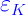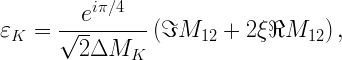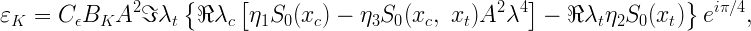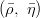## Constraint fromIndirect CP violation in the Kaon system is usually expressed in terms ofparameter which is the fraction of the CP violating component in the mass eigenstates and which is usually defined as:whereTop and charm quarks contribute to the expression of the mixing in K0-K0 system. The calculation of the box diagram giveswherewhich allows one to writewhereThe expression actually used in the UT fit is obtained by writingin terms ofand the other elements of CKM matrix:The experimental values we use are summarized in the Table of Inputs. The representation of this constraint in theplane is given below.EPS - PDF - PNG - JPG - GIF

Ideas, requests, problems regarding this web site? Send feedback